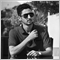# Help me to use indicator inside EA as a function19

Hi everybody,

I'm wondering if there is a way to convert DT oscillator (attached) to a function, such as this:

double calculateDT(int timeframe, int PeriodRSI, int PeriodStoch,int PeriodSK, int PeriodSD,int MAMode,int line,int shift)

{

//

//here is the codes

//

}

here is the main "start" section of indicator:

```int start()
{
int i,limit;
int counted_bars = IndicatorCounted();

if(counted_bars < 0) return(-1);
if(counted_bars > 0) counted_bars--;
limit=Bars-counted_bars;

if (returnBars) { SK = limit; return(0); }
if (timeFrame != Period())
{
limit = MathMax(limit,MathMin(Bars,iCustom(NULL,timeFrame,IndicatorFileName,"getBarsCount",0,0)*timeFrame/Period()));

for(i=0; i<limit; i++)
{
int y = iBarShift(NULL,timeFrame,Time[i]);
SK[i] = iCustom(NULL,timeFrame,IndicatorFileName,"",PeriodRSI,PeriodStoch,PeriodSK,PeriodSD,MAMode,0,y);
SD[i] = iCustom(NULL,timeFrame,IndicatorFileName,"",PeriodRSI,PeriodStoch,PeriodSK,PeriodSD,MAMode,1,y);
}
return(0);
}

for(i=limit-1; i>=0; i--)
{
RSI[i] = iRSI(NULL,0,PeriodRSI,PRICE_CLOSE,i);
double LLV = RSI[ArrayMinimum(RSI,PeriodStoch,i)];
double HHV = RSI[ArrayMaximum(RSI,PeriodStoch,i)];
if ((HHV-LLV)!=0)
StoRSI[i] = 100.0*((RSI[i] - LLV)/(HHV - LLV));
else  StoRSI[i] = 0;
}
for(i=limit; i>=0; i--) SK[i]=iMAOnArray(StoRSI,0,PeriodSK,0,MAMode,i);
for(i=limit; i>=0; i--) SD[i]=iMAOnArray(    SK,0,PeriodSD,0,MAMode,i);
return(0);
}```

//

//

//

I almost did it and works on current time frame, but it's not working on different timeframes. Would somebody please help me???

Files:24689

mst_ab: I'm wondering if there is a way to convert DT oscillator (attached) to a function, such as this:
`double calculateDT(int timeframe, int PeriodRSI, int PeriodStoch,int PeriodSK, int PeriodSD,int MAMode,int line,int shift)`

That is exactly what you should write, an encapsulated iCustom call to make your code self-documenting.
Detailed explanation of iCustom - MQL4 and MetaTrader 4 - MQL4 programming forum19

whroeder1:

That is exactly what you should write, an encapsulated iCustom call to make your code self-documenting.
Detailed explanation of iCustom - MQL4 and MetaTrader 4 - MQL4 programming forum

Thanks whroeder1 for your reply, but I don't want to use iCustom because it's very slow and my EA calls the function several times in each tick, so EA optimization will be almost impossible. I want to import the calculation of indicator inside my own function...